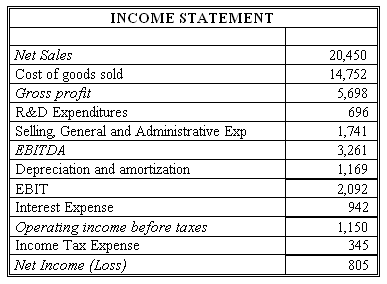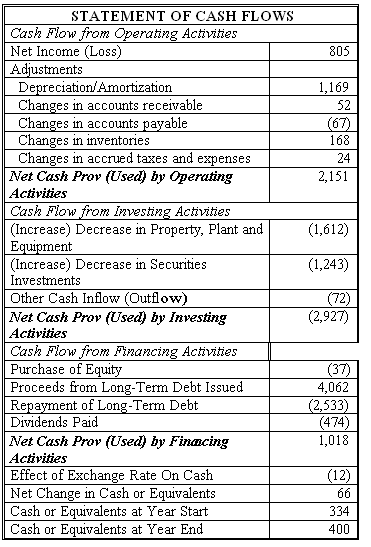### CFA Practice Question

There are 534 practice questions for this study session.

### CFA Practice Question

Connie's Sporting Goods (CSG) has net income of \$805 million for 2016. Using information from the CSG's financial statements below, use the CFO approach to find what FCFF and FCFE should be for CSG. Assume the income tax rate is 30%.Correct Answer: \$1,198 million and \$2,068 million

FCFF = CFO + Int (1 - Tax rate) - FCInv
= 2151 + 942 (1 - 0.3) - 1612 = \$1,198 million

FCFE = CFO + Net borrowing - FCInv
= 2151 + (4062 - 2533) - 1612 = \$2,068 million